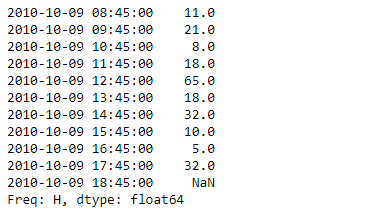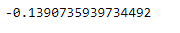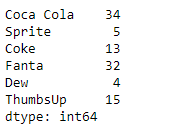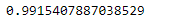# Python | Pandas Series.autocorr()

• Last Updated : 17 Feb, 2019

Pandas series is a One-dimensional ndarray with axis labels. The labels need not be unique but must be a hashable type. The object supports both integer- and label-based indexing and provides a host of methods for performing operations involving the index.

Pandas` Series.autocorr()` function compute the lag-N autocorrelation. This method computes the Pearson correlation between the Series and its shifted self.

Syntax: Series.autocorr(lag=1)

Parameter :
lag : Number of lags to apply before performing autocorrelation.

Returns : float

Example #1: Use `Series.autocorr()` function to compute the lag-N auto-correlation of the underlying data for the given series object.

 `# importing pandas as pd ` `import` `pandas as pd ` ` `  `# Creating the Series ` `sr ``=` `pd.Series([``11``, ``21``, ``8``, ``18``, ``65``, ``18``, ``32``, ``10``, ``5``, ``32``, ``None``]) ` ` `  `# Create the Index ` `index_ ``=` `pd.date_range(``'2010-10-09 08:45'``, periods ``=` `11``, freq ``=``'H'``) ` ` `  `# set the index ` `sr.index ``=` `index_ ` ` `  `# Print the series ` `print``(sr) `

Output :Now we will use `Series.autocorr()` function to compute the lag-n auto-correlation of the underlying data for the given series object.

 `# return the auto correlation ` `result ``=` `sr.autocorr() ` ` `  `# Print the result ` `print``(result) `

Output :As we can see in the output, the `Series.autocorr()` function has successfully returned the auto correlation of the underlying data of the given series object by lag 1.

Example #2 : Use `Series.autocorr()` function to compute the lag-N auto-correlation of the underlying data for the given series object. Take the lag value equal to 3.

 `# importing pandas as pd ` `import` `pandas as pd ` ` `  `# Creating the Series ` `sr ``=` `pd.Series([``34``, ``5``, ``13``, ``32``, ``4``, ``15``]) ` ` `  `# Create the Index ` `index_ ``=` `[``'Coca Cola'``, ``'Sprite'``, ``'Coke'``, ``'Fanta'``, ``'Dew'``, ``'ThumbsUp'``] ` ` `  `# set the index ` `sr.index ``=` `index_ ` ` `  `# Print the series ` `print``(sr) `

Output :Now we will use `Series.autocorr()` function to compute the lag-n auto-correlation of the underlying data for the given series object.

 `# return the auto correlation ` `# by lag-3 ` `result ``=` `sr.autocorr(lag ``=` `3``) ` ` `  `# Print the result ` `print``(result) `

Output :As we can see in the output, the `Series.autocorr()` function has successfully returned the auto correlation of the underlying data of the given series object by lag 1.

My Personal Notes arrow_drop_up
Recommended Articles
Page :